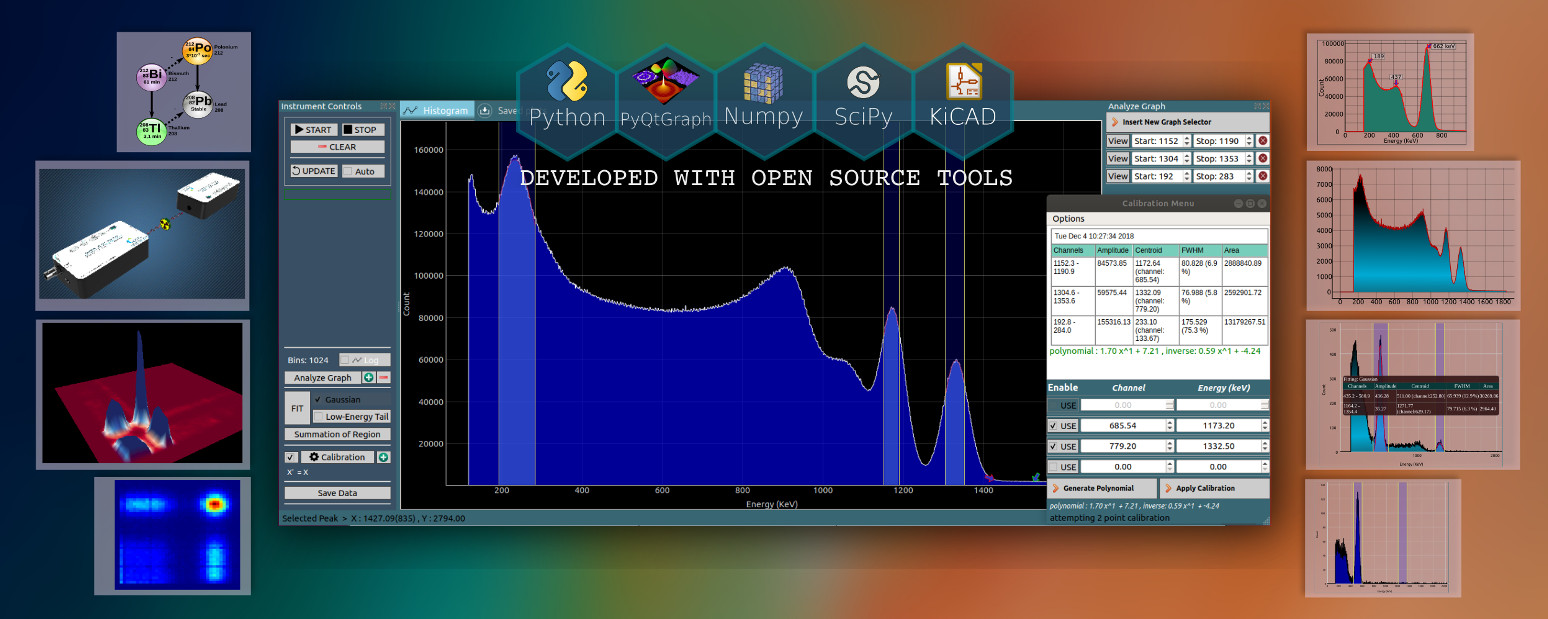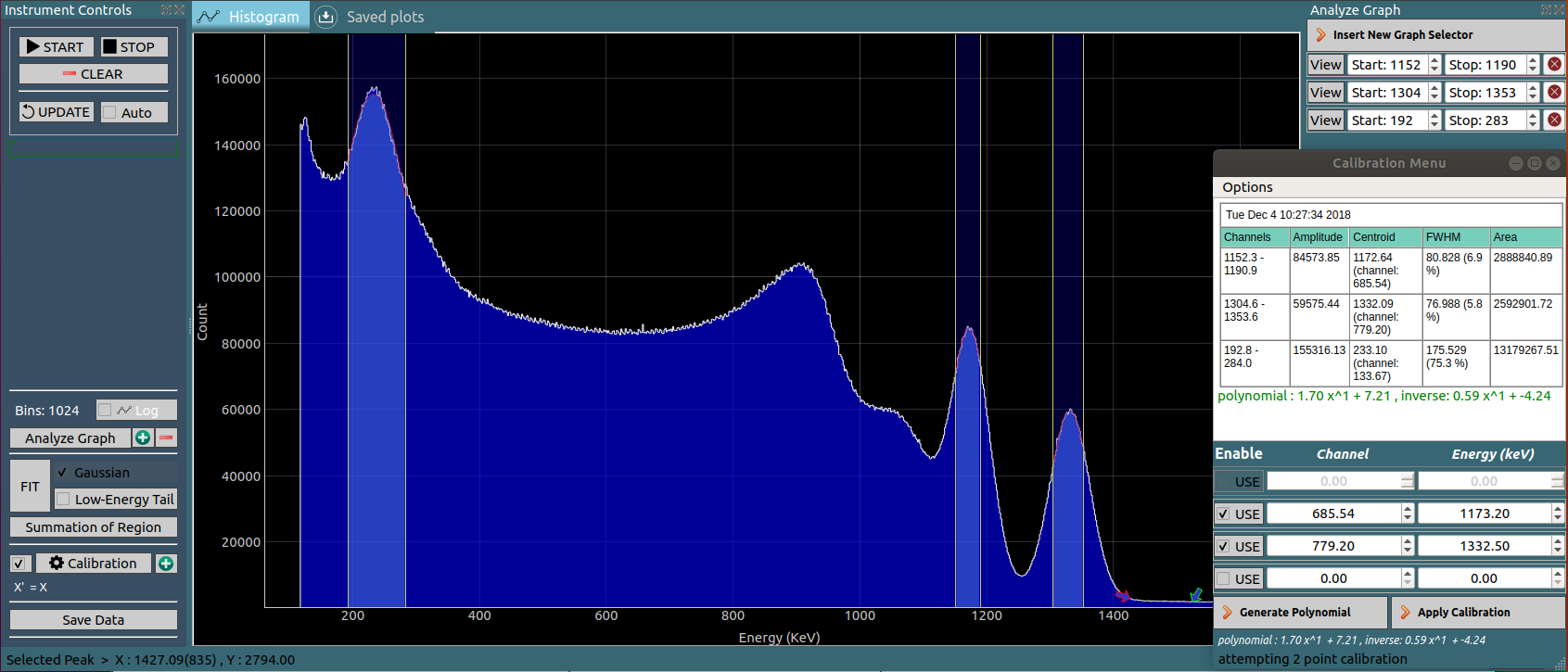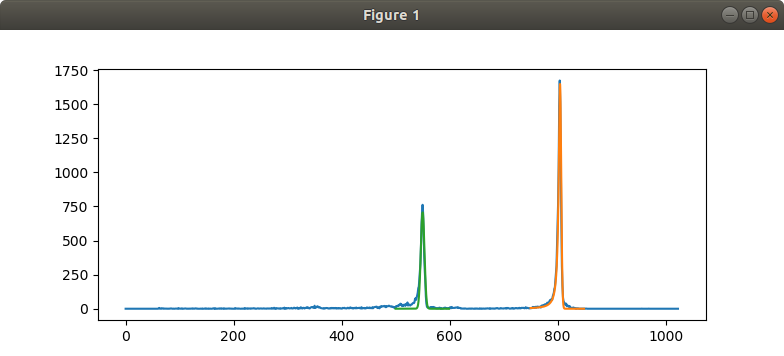# CNSPEC

## — Software for nuclear data acquisition and analysis —Tested on Ubuntu 18.04 and Windows 7/10

## MAIN FEATURES

+

Compatible with our Multi Channel analyzers, Alpha Spectrometers, and Gamma Spectrometers

Analysis -> multi-point calibration, Gaussian Fitting, Low-Energy tail fitting, summation

Offline Data analysis of csv files

LGPL licensed source code supplied free with the instrument

Standalone Python library for acquisition and analysis.Spectrum shown was acquired with our Gamma Spectrometer(gammaspec1k) and a Cobalt-60 source. Regions have been inserted to calibrate the instrument using the known gamma energies, and centroids which were estimated using gaussian curve fitting.

#### Calibration PageThis tutorial explains how to use the region selectors for calculating centroids through gaussian fitting, and using the values to calibrate the instrument.

### Using the Python library

CNSPEC version > 6.0.0 must be installed. Or download the library : MCALib.py, and make sure the following packages are installed

• python3
• python3-numpy
• python3-scipy
• matplotlib

### Capture data from an instrument and plot it: example.py

Create a new python file. In case you downloaded MCALib.py , it must be located in the same directory.

• Initialization
``````import time,sys
from MCALib import connect

device = connect(autoscan = True) # automatically detect the hardware
#device = connect(port = '/dev/ttyUSB0')  #Search on specified port
``````
• Check if successfully connected
``````if not device.connected:
sys.exit(0)
``````
• Start acquiring data
``````print("Device Version",device.version,device.portname) #Display the version number

device.startHistogram()  #start data acquisition
time.sleep(5) # Wait for 5 seconds for gather some events.
``````
• Retrieve data from the hardware, and plot it
``````device.sync() # fetch data from the hardware
x = device.getHistogram()  # Array of counts

from matplotlib import pyplot as plt
plt.plot(x)
plt.show()
``````

### Offline data analysis: offline_example.py

Create a new python file. In case you downloaded MCALib.py , it must be located in the same directory.

## Results from offline data analysis

+

The results of the above code on offline analysis of 212-Bismuth spectrum are shown

Gaussian fitting was carried out on the first peak(Green) and overlaid.

Gaussian+Low energy tail(Lorentzian) was carried out on the second peak (Orange).The Python library is used to encourage students to understand the acquired data, and interpret and analyze it manually instead of relying on the graphical interface. However, the GUI is far more efficient, and feature rich.
• Initialization
``````import time,sys
import numpy as np
from MCALib import connect

dev = connect()  # 'dev' contains a range of methods for acquisition and analysis
``````
• Load data from a text file(csv, txt, dat…)
``````fname = 'bi212.csv'  #Supply your filename here.
# Get the data. Supply an optional name argument in case of multiple files/connected hardware.
x = dev.getHistogram() #name = fname / name='/dev/ttyUSB0'
``````
• Display its contents. ( optional step )
``````np.set_printoptions(threshold = np.inf,precision = 0,suppress=True) #print the whole array. No decimal Points. Suppress scientific notation
print (x)
``````
• Plot the spectrum with matplotlib
``````import matplotlib.pyplot as plt
plt.plot(x) #Plot RAW data
``````
• Attempt a gaussian fit around the first peak located between channels 500 and 600
``````FIT = dev.gaussianFit([500,600]) #Apply a gaussian FIT between 500 and 600 channel.
if FIT: #If fit was successful
plt.plot(FIT['X'],FIT['Y']) #Plot fitted data
print('Gaussian Fit : ',FIT['centroid'],FIT['fwhm'])
``````
• Attempt a Gaussian+Low-energy Tail(Lorentzian) fit around the second peak located between channels 750 and 850
``````FIT = dev.gaussianTailFit([750,850]) #Apply a gaussian+Lorentzian FIT between 700 and 900 channel.
if FIT:
plt.plot(FIT['X'],FIT['Y']) #Plot fitted data
print('Gaussian+low energy tail Fit : ',FIT['centroid'],FIT['fwhm'])
``````
• Show the matplotlib window
``````plt.show()
``````

## Future goals

We are in the process of implementing a flow based approach for numerical analysis. A video is shown below.

List mode data can be passed through which are basically operators for binning/analysis/fitting etc, and can be further attached to visualization blocks such as 2D and 3D histograms.

Although the same can be achieved with 10 lines of Python code, this approach might come in handy for demonstrating the flow of logic for processing data. There is a long way to go, and we have also envisioned energy gates for processing multi parameter list mode data.